# Grasshopper series of articles-understanding basic data types

This list is all about the basic parameter types (there is no specific shape of data, such as integers). It takes some time for everyone to memorize these parameter types.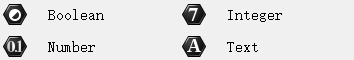Boolean：

Boolean value indicates two states of yes or no, such as judging whether the point is online, whether the curve is closed, etc.

Integer：

Let the input data output as integers, the grasshopper algorithm shown in the figure below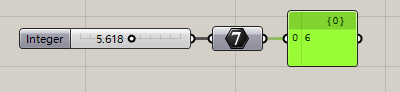Number：

Floating point number

Text：Text dataColour：Output RGB color values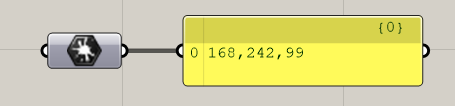Complex：

Composite number, general output value range

Culture：

Domain:

Data Range

Domain²：

Data Range of suface

Guid：

Get the ID number of the object

Matrix：

Get the Matrix Data

Time：

Get the time dataData：

Get all data types, whether they are points, lines, numbers, boolean values, etc., can be input into this calculator, an example is shown in the figure below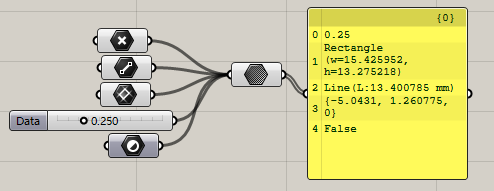Data Path：

Data path is a very, very important concept in Grasshopper, which will be introduced in detail in later courses, here is a brief understanding of what is data path.

The character pointed by the arrow in the figure below is the data path {0} means that the following data are all under the path 0 {0; 0; 1} means that the data of 1 is under the path {0; 0; 1}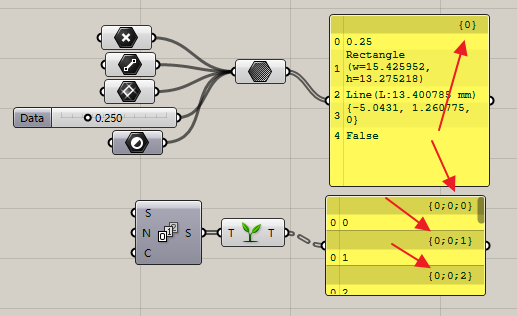File Path：

The function is to obtain some external documents such as rhino files or some table files.

Shader：

Scroll to Top